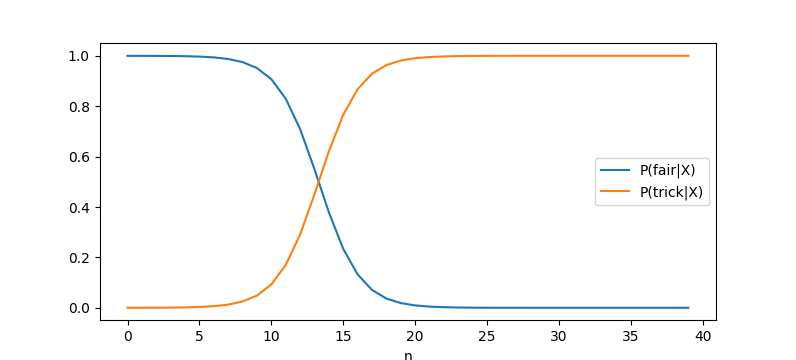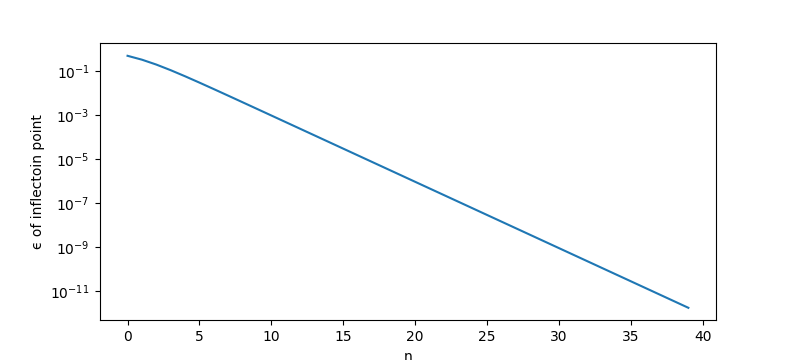# How to compute the probability you are flipping a trick coin

by lsusr1 min read15th May 20218 comments

# 18

Suppose you flip a coin times and get heads in a row. What is the probability the next flip will land heads?

Suppose the coin is either a fair coin with one heads or a trick coin with two heads. Let denote our training data of heads. We want to find and . Let . It follows that .

We use Bayes theorem .

I have flipped perhaps a hundred coins. One was double-headed trick coin. On the one hand, I flip trick coins with anomalously high frequency. On the other hand, double-headed trick coins are more likely than regular coins to get flipped than fair coins. I estimate the flip frequency of double-headed trick coins to be one in ten thousand.

What does it look like when we graph our probabilities with ?For the first 5 heads you can remain confident you are flipping a regular coin. Around 10 heads the exponential takes off. You quickly become confident you are not flipping a regular coin. At 20 heads in a row you can be confident you are not flipping a regular coin.

# The Inflection Point

The inflection point occurs when the probabilities are equal.A linear increase in your data has predictive power equal to an exponential increase in the strength of your prior.

8 comments, sorted by Highlighting new comments since
New Comment

The exponential is because updates happen on a logarithmic scale. Do you have a simple variant of the problem in mind where we don't get exponentials? When I try to construct one, I have to start from "we don't get exponentials" and calculate how the probabilities of different hypotheses would have to converge over time.

2^-n is in fact the probability of a coin showing n heads. Where is the choice?

If you're interested in making a follow-up post, I'd enjoy an analysis of the possibilities when the coin is not fair but is also not double sided. For example, if a coin has a 75% chance of turning up heads, how does the probability look? If a coin turns up heads 50 times in a row, it's probably neither fair nor a 75/25 coin, but if it turns up heads 10 times in a row I might guess it to be 75/25.

If you’re interested in making a follow-up post, I’d enjoy an analysis of the possibilities when the coin is not fair but is also not double sided. For example, if a coin has a 75% chance of turning up heads, how does the probability look?

I wrote this! The graphs of P(bias|flips) are fun. See this post starting at "computing a credible interval":

https://justinpombrio.net/2021/02/19/confidence-intervals.html

Sorry if you're viewing on mobile, I need to fix my styling.

A string of all-heads makes "the coin always flips heads" more likely than any other option, given equal priors, no matter how long the string is. So, what is your prior distribution of bias for "a coin someone tells you to flip"? I'd say 1000:10:1:.001 for fair:biased a tiny but detectable amount:always heads:any other bias amount

I've read that it's not possible to bias a coin - you can bias a coin toss if you know which way up it starts, but the coin itself will always be fair. But I confess that I don't know what assumptions they were making, so for all I know you could make something that would be recognizably a coin but that analysis wouldn't apply.

If one side is heavier, it will land that side down more often. You can see this with a household experiment of gluing a quarter to a circle of cardboard the same thickness, and then flipping it.

So I was thinking of this paper (pdf), which I misremembered somewhat - you can't make a coin biased for "toss and catch", but you can make it biased for "toss and let it bounce". (And for "spin on a table".) Given that, "can't bias a coin" is probably too strong, though it's in the title of the paper.

Props for suggesting an actual experiment! I didn't feel like doing it though :p